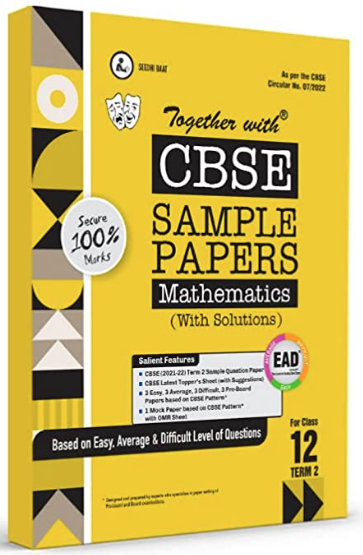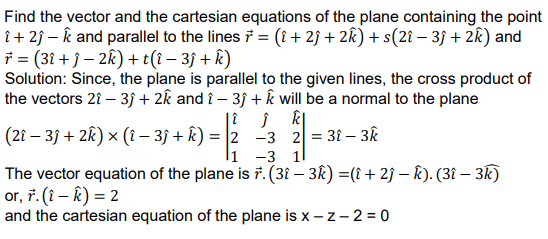# Find the vector and the cartesian equations of the plane containing the point 𝑖̂+ 2𝑗̂− 𝑘̂ and parallel to the lines 𝑟⃗ = (𝑖̂+ 2𝑗̂+ 2𝑘̂) + 𝑠(2𝑖̂− 3𝑗̂+ 2𝑘̂) and 𝑟⃗ = (3𝑖̂+ 𝑗̂− 2𝑘̂) + 𝑡(𝑖̂− 3𝑗̂+ 𝑘̂)

CBSE Sample Question Paper, Class 12 Mathematics Term 2 Question - Find the vector and the cartesian equations of the plane containing the point 𝑖̂+ 2𝑗̂− 𝑘̂ and parallel to the lines 𝑟⃗ = (𝑖̂+ 2𝑗̂+ 2𝑘̂) + 𝑠(2𝑖̂− 3𝑗̂+ 2𝑘̂) and 𝑟⃗ = (3𝑖̂+ 𝑗̂− 2𝑘̂) + 𝑡(𝑖̂− 3𝑗̂+ 𝑘̂)## Find the vector and the cartesian equations of the plane containing the point 𝑖̂+ 2𝑗̂− 𝑘̂ and parallel to the lines 𝑟⃗ = (𝑖̂+ 2𝑗̂+ 2𝑘̂) + 𝑠(2𝑖̂− 3𝑗̂+ 2𝑘̂) and 𝑟⃗ = (3𝑖̂+ 𝑗̂− 2𝑘̂) + 𝑡(𝑖̂− 3𝑗̂+ 𝑘̂)